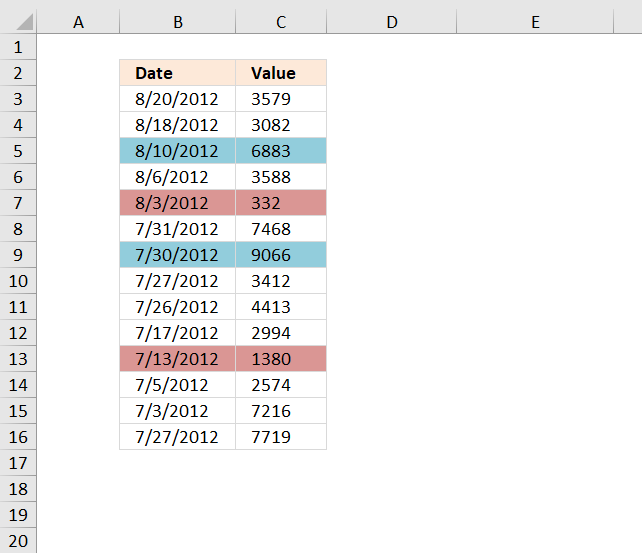Author: Oscar Cronquist Article last updated on November 30, 2018The image above shows rows highlighted based on value in column C being the largest or smallest in that particular month.

Conditional formatting formula (Max)

=MAX(IF((YEAR(\$B\$3:\$B\$16)= YEAR(\$B3))* (MONTH(\$B\$3:\$B\$16)=MONTH(\$B3)), \$C\$3:\$C\$16, ""))=\$C3

Conditional formatting formula (Min)

=MIN(IF((YEAR(\$B\$3:\$B\$16)= YEAR(\$B3))* (MONTH(\$B\$3:\$B\$16)=MONTH(\$B3)), \$C\$3:\$C\$16, ""))=\$C3

### Explaining CF formula (Max)

Step 1 to 3 make sure that values from the correct year and month are extracted based on the date of the current row.

#### Step 1 - Compare year to dates

The YEAR function returns the year from an Excel date.

YEAR(\$B\$3:\$B\$16)= YEAR(\$B3))

becomes

YEAR({41141; 41139; 41131; 41127; 41124; 41121; 41120; 41117; 41116; 41107; 41103; 41095; 41093; 41117})= YEAR(41141))

and returns

{TRUE; TRUE; TRUE; TRUE; TRUE; TRUE; TRUE; TRUE; TRUE; TRUE; TRUE; TRUE; TRUE; TRUE}.

#### Step 2 - Compare month to dates

The MONTH function returns a number representing the month from an Excel date.

MONTH(\$B\$3:\$B\$16)=MONTH(\$B3)

becomes

MONTH({41141; 41139; 41131; 41127; 41124; 41121; 41120; 41117; 41116; 41107; 41103; 41095; 41093; 41117})=MONTH(41141)

and returns

{TRUE; TRUE; TRUE; TRUE; TRUE; FALSE; FALSE; FALSE; FALSE; FALSE; FALSE; FALSE; FALSE; FALSE}.

#### Step 3 - Multiply arrays

Both conditions must be met in order to extract the number, we apply AND-logic if we multiply the arrays.

(YEAR(\$B\$3:\$B\$16)= YEAR(\$B3))* (MONTH(\$B\$3:\$B\$16)

becomes

{TRUE; TRUE; TRUE; TRUE; TRUE; TRUE; TRUE; TRUE; TRUE; TRUE; TRUE; TRUE; TRUE; TRUE}*{TRUE; TRUE; TRUE; TRUE; TRUE; FALSE; FALSE; FALSE; FALSE; FALSE; FALSE; FALSE; FALSE; FALSE}

and returns

{1;1;1;1;1;0;0;0;0;0;0;0;0;0}.

#### Step 4 - Convert boolean values

The IF function returns row number if the logical expression evaluates to TRUE and nothing "" if FALSE.

IF((YEAR(\$B\$3:\$B\$16)= YEAR(\$B3))* (MONTH(\$B\$3:\$B\$16)=MONTH(\$B3)), \$C\$3:\$C\$16, "")

becomes

IF({1;1;1;1;1;0;0;0;0;0;0;0;0;0}, \$C\$3:\$C\$16, "")

and returns

{3579; 3082; 6883; 3588; 332; ""; ""; ""; ""; ""; ""; ""; ""; ""}.

#### Step 5 - Get largest number from array

The MAX function returns the maximum value from a cell range or array.

MAX(IF((YEAR(\$B\$3:\$B\$16)= YEAR(\$B3))* (MONTH(\$B\$3:\$B\$16)=MONTH(\$B3)), \$C\$3:\$C\$16, ""))

becomes

MAX({3579; 3082; 6883; 3588; 332; ""; ""; ""; ""; ""; ""; ""; ""; ""})

and returns 6883.

#### Step 6 - Compare the largest value to current value

The equal sign compares the values and returns TRUE if they match.

MAX(IF((YEAR(\$B\$3:\$B\$16)= YEAR(\$B3))* (MONTH(\$B\$3:\$B\$16)=MONTH(\$B3)), \$C\$3:\$C\$16, ""))=\$C3

becomes

6883=3579

and returns FALSE. Row 3 is not highlighted.